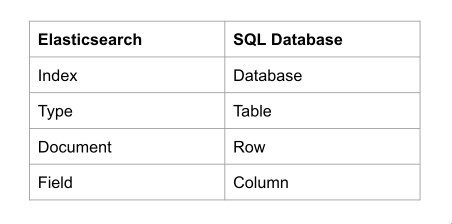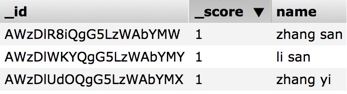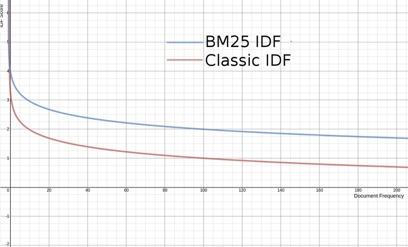# ElasticSearch 简介

## 为什么需要使用 ES 进行搜索

### 结构化数据 VS 非结构化数据

• 结构化数据：
也称作行数据，关系型数据库进行存储和管理,是由二维表结构来逻辑表达和实现(可以使用行、列来表现)的数据，严格地遵循数据格式与长度规范。
• 非结构化数据：
又可称为全文数据，不定长或无固定格式，不适于由数据库二维表来表现，包括所有格式的办公文档、XML、HTML、word文档，邮件，各类报表、图片和音频、视频信息等。## ES 基本概念介绍

### ES 存储模型

ES 在设计存储模型时，考虑了大家从关系型数据库转换肯能带来的困难，于是设计了 Index、Type、Document、Field 分别于对应传统关系型数据库(比如 MySQL) 的 Database、Table、Row、Column。### ES 与 Lucene

ES 底层基于 Lucene 开发，Lucene作为其核心来实现索引搜索的功能。我们虽然讲的是 ES，但很大一部分内容是 Lucene 的实现。

# 评分机制

## ES 相似度(Similarity)机制

### 什么是相似度？

ES 把每一篇文档与查询词的相似度计算出来，使用一个数值来表示（评分），然后按照评分又高到底的顺序返回前 n 条数据。这就是一次查询的大致过程。

### 如何计算相似度？

$score(q,d)= \displaystyle \sum^{}_{t\, in \, d} {(tf(t \, in \, d) \cdot idf(t)^2 \cdot norm(t , d) \cdot boost(t) )}$

### Lucene 评分函数的产生

Lucene 评分函数借鉴了词频/逆向文档频率（TF/IDF:term frequency/inverse document frequency）算法 和 向量空间模型（VSM:vector space model）

## 词频/逆向文档频率（TF/IDF）

### 公式详解：

$TF score * IDF score * fieldNorms$

$TF score$ 是词频得分，它的公式是:

$\sqrt{tf}$

tf 是要查询的词在一个文档中出现的次数。

$IDF score$ 是逆向文档频率的得分，等于文档频率 的倒数取log。

$idf(t\,in\,d)=1+\log{_2 2} \frac{numDocs}{docFreq+1}$

numDocs 即为文档总数； docFreq 即为出现要查询词出现在文档(这个概念对应关系型数据库的“记录”,见上文)中出现的次数；
IDF score 的意义在于考虑查询的词在文档总数出现的频率，对总分产生影响。查询的词在文档中出现的频率越高，对结果产生的影响越小。比如想 “的”，“你” 等常见的词几乎出现在每一个文档中，对结果会有很小的影响。

$fieldNorms$ 是字段长度归一值。它的计算方式为：

$\frac{1}{\sqrt{length}}$

length 表示文档的长度。
fieldNorms 的意义在于考虑文档长度对于查询得分的影响。例如在一条短信中匹配到搜索的词与在一本书中匹配到，肯定是这个短信更有可能属于结果集。

$TF score * IDF score * fieldNorms$

$= \sqrt{tf} * （1+\log{_2 2} \frac{numDocs}{docFreq+1}） * \frac{1}{\sqrt{length}}$

### TF/IDF 在 ES 中的体现

ES 中的数据：## 空间向量模型 (VSM)

I am happy in summer 。
After Christmas I’m a hippopotamus 。
The happy hippopotamus helped Harry 。$cos{\theta}= \frac{\vec{a} \cdot \vec{b}}{|a|\cdot |b|}$

## Lucene 实用评分函数

Lucene 实用评分函数是有余弦相似度公式演变而来。图中相同颜色的框代表的含义是一样的。$queryBoost(q)$$docBoost(d)$ 分别代表查询的权重提升和文档的权重提升，可以更为灵活的手工指定，影响搜索结果。

## BM25 相似度

BM25 全称 Okapi BM25 是 Okapi Best Match 25 的缩写。
ES 版本 5.0 将 TF/IDF 模型更换为了 BM25 模型。BM25可以看做是 TF/IDF 的改良。
BM25 与 TF/IDF 相比，主要体现在 TF 和 fieldNorms 的计算上。

### IDF### TF

TF 值收敛到一个值($k$)。在一定程度上，词频越高，固然得分也应该越高。但是不能没有限度。否则像是网页搜索，站长可以通过作弊，使得词频达到极高的值，就达到了垄断这个词的效果 了。### fieldNorms

BM25 将 fieldNorms 与词频进行合并，一起对得分产生影响,称为 tfNorm

### tfNorm

$tfNorm = ((k + 1) * tf) / (k * (1.0 - b + b * L) + tf)$

$L$：表示文档的长度是平均文档长度的多少倍。计算方式：$\frac{|d|}{avgDl})$
$b$ 参数的作用：调节 L 的影响程度,当b=0时，文档的长度对于结果没有影响### BM25 公式

$IDF * ((k + 1) * tf) / (k * (1.0 - b + b * (\frac{|d|}{avgDl})) + tf)$

# 扩展阅读

1. 来自 ElasticSearch 权威指南：https://www.elastic.co/guide/cn/elasticsearch/guide/current/scoring-theory.html ↩︎